International
Tables for
Crystallography
Volume B
Reciprocal space
Edited by U. Shmueli

International Tables for Crystallography (2006). Vol. B. ch. 2.5, p. 318   | 1 | 2 |

## Section 2.5.6.6. The algebraic and iteration methods

B. K. Vainshteinc

#### 2.5.6.6. The algebraic and iteration methods

| top | pdf |

These methods have been derived for the two-dimensional case; consequently, they can also be applied to three-dimensional reconstruction in the case of orthoaxial projection.

Let us discretize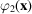by a net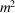of points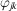; then we can construct the system of equations (2.5.6.10).

When h projections are available the condition of unambiguous solution of system (2.5.6.10)is: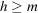. At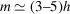we can, in practice, obtain sufficiently good results (Vainshtein, 1978).

Methods of reconstruction by iteration have also been derived that cause some initial distribution to approach onesatisfying the condition that its projection will resemble the set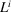. Let us assign on a discrete netas a zero-order approximation the uniform distribution of mean values (2.5.6.7)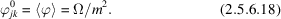The projection of the qth approximation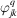at the angle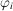(used to account for discreteness) is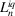.

The next approximation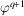for each point jk is given in the method of `summation' by the formula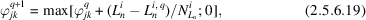where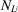is the number of points in a strip of the projection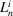. One cycle of iterations involves runningaround all of the angles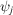(Gordon et al., 1970).

When carrying out iterations, we may take into account the contribution not only of the given projection, but also of all others. In this method the process of convergence improves. Some other iteration methods have been elaborated (Gordon & Herman, 1971; Gilbert, 1972b; Crowther & Klug, 1974; Gordon, 1974).

### ReferencesCrowther, R. A. & Klug, A. (1974). Three dimensional image reconstruction on an extended field – a fast, stable algorithm. Nature (London), 251, 490–492.Google ScholarGilbert, P. F. C. (1972b). Iterative methods for the three-dimensional reconstruction of an object from projections. J. Theor. Biol. 36, 105–117.Google ScholarGordon, R. (1974). A tutorial on ART (algebraic reconstruction techniques). IEEE Trans. Nucl. Sci. NS-21, 78–93.Google ScholarGordon, R. & Herman, G. T. (1971). Reconstruction of pictures from their projections. Commun. ACM, 14, 759–768.Google ScholarVainshtein, B. K. (1978). Electron microscopical analysis of the three-dimensional structure of biological macromolecules. In Advances in optical and electron microscopy, Vol. 7, edited by V. E. Cosslett & R. Barer, pp. 281–377. London: Academic Press.Google Scholar